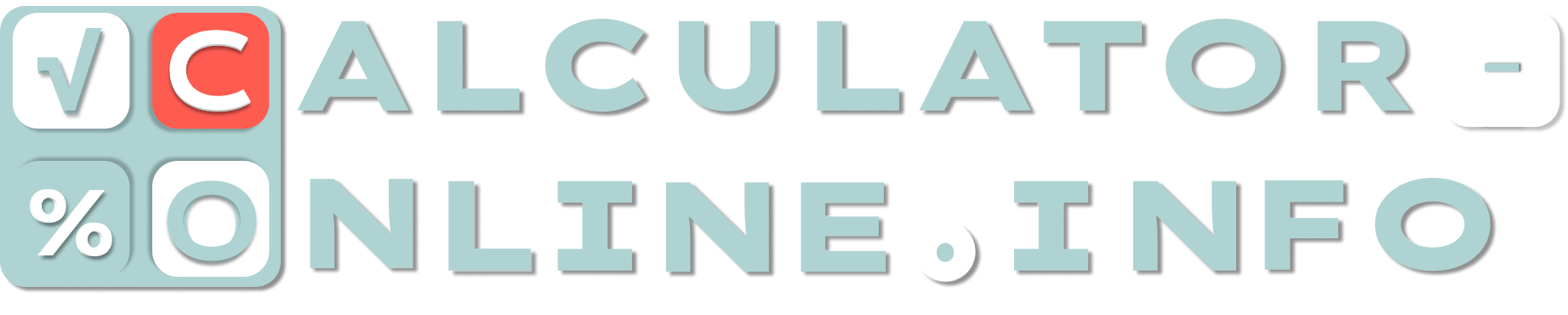# Reverse percentage calculator | How to reverse percentage?

Of what number
is
%
=
0.00
×

This is the calculator which solves the tasks with reverse percentage. It determines the initial number when only the percentage of it is known.

## How to reverse percentage?

There are several methods to calculate reverse percentage. Let’s use an example when we know that 48% of unknown value is 300.

### Classic calculation

This method of calculation means that in the beginning you should find out the value of 1%. To do this it is necessary to divide a known number by a percentage. (300 / 48) = 6.25, which is equal to 1 percent of unknown value. Then you should multiply this number by 100. Due to our example it will be 625. As a conclusion, 300 is 48% of 625.

The inverse percentage formula looks like that:

### Unknown value = (Number / Percentage) × 100

Cross to cross calculation

How to calculate reverse percentage in another method? According to the second approach, the task must be represented in the form of two equations:

Unknown value = 100%

300 = 48%

It is necessary to write them in two columns, mentally draw a cross, and multiply the numbers standing across (300 × 100 = 30 000). The resulting number must be divided by the value not used in the multiplication (30 000 / 48 = 625).

The full formula for calculating inverse percentage will be so:

Unknown value = (Number × 100) / Percentage

### How reverse percent calculator works?

Inverse percentage calculator makes all calculation itself. To know what the original number is, you should only enter the known values. The result will appear immediately.

Rating
( 4 assessment, average 4.75 from 5 )Mathematician/ author of the article